### IMO Shortlist 1987 problem 15

Kvaliteta:
Avg: 0.0
Težina:
Avg: 0.0
Let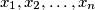$x_1,x_2,\ldots,x_n$ be real numbers satisfying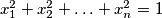$x_1^2+x_2^2+\ldots+x_n^2=1$. Prove that for every integer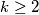$k\ge2$ there are integers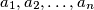$a_1,a_2,\ldots,a_n$, not all zero, such that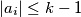$|a_i|\le k-1$ for all$i$, and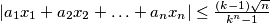$|a_1x_1+a_2x_2+\ldots+a_nx_n|\le{(k-1)\sqrt n\over k^n-1}$. (IMO Problem 3)

Proposed by Germany, FR
Source: Međunarodna matematička olimpijada, shortlist 1987